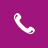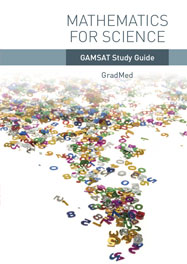020 7584 5818# Mathematics For Science

## Syllabus

• Percentages and percentage calculations
• Algebra and equations
• Changing the subject of an equation
• What an equation tells us
• Indices and calculations involving indices
• Standard form and conversions involving standard form
• Physical quantities in science
• Converting units
• Finding units of a new term
• Scale factors
• Volumes and areas
• Basic trigonometry
• Proportionality in graphs
• Inverse proportionality
• Straight line graphs, gradients and intercepts
• Power law graphs and equations
• Curved graphs
• Logarithms and the log scale
• Natural logs
• Examples of using log scales
• Exponential equations
• Linearising equations
• Further examples
• Half-Life
• The mathematics of radioactive and exponential decay

Other mathematical skills are introduced through analysis of sample questions during the course